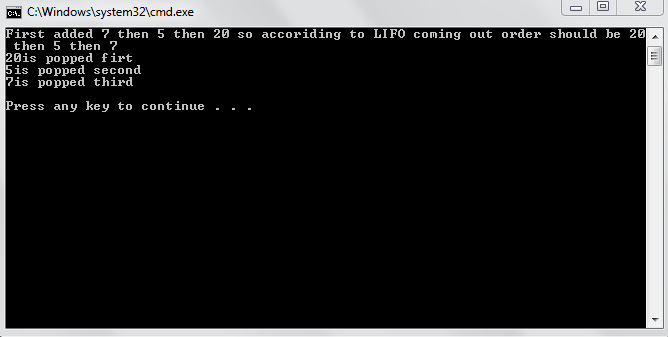# Introduction to Stacks

In this tutorial, you will 1. Learn the concept of stacks. 2. Be given practical examples of LIFO 3. Learn what the types of implementation of stack are? 4. learn the array based implementation of stacks. What is the concept of stacks? The concept of stacks is very simple. It is that if you insert an element in a stack, it always occupies top position and when you remove element, the element at top is always removed. i.e. the element inserted last is removed first. Another name given to such insertion is LIFO i.e. last in first out. In stacks, elements can only be inserted and removed from one end. Thus, if in a linked list, if elements are added and removed from end or start but not both(only end or only start ) it will act as a stack . Implementation of stacks will be discussed later. What are some practical examples of LIFO? There are many examples of LIFO (Last in First out) in practical life which will help you understand stacks better . 1. Putting dishes on each other. When you put dish for washing or any other purpose, basically it is like stacks. You always put the new dish on top and while removing, you remove the top most dish. 2. For all the people with accounting background, you would know that the concept of LIFO is also used in accounting. When items are added in an inventory, the item added last is sold first. What are different implementations of stacks ? There are two types of implementations of stacks 1. Array based implementation. 2. Pointer (linked list) based implementation. What is array based implementation of stacks? In array based implementation, we use arrays to store data and we add new data in arrays and delete data from array. Actually a counter is used to have the information of number of elements filled in array and while adding data, counter is incremented and while removing data, counter’s value is decreased. It is mostly used when you know the maximum amount of data. C++ code and step by step implementation of code is given.
`class Stack {private:	char arr;	int top;public:	Stack():top(0){}	void push(char value)	{		arr[top]=value;		top++;	}	int get_top()	{		if(Empty())		{			return -1;		}		return arr[top-1];	}	bool Empty()	{		if(top==0)			return 1;		return 0;	}	int pop ()	{		top--;		return arr[top];	} };`
Here in class a 50 element stack is made assuming that maximum number of elements will be 50. If you don’t know the maximum number of elements, for array based implementation, a very big array is made but space is wasted. A counter top is also made. While initializing, top is given value zero . When we add data, if value of top is zero data is entered at first location of array and so on. After insertion of data, top is incremented. So that while inserting next element, data will be inserted in next vacant location. Pop function is used to just delete last element and also return it. Note we have first top—and then return command because initially top was pointing to next element it must be first decremented so that last element will be returned. Also, after decrement, when we add a new element, it will be added in place of deleted element and hence, in actual, we over write the deleted element. The empty() only checks if stack is empty and will return 1. if stack is actually empty. The gettop() function just returns the value of top. Do go through the code to understand usage of stacks. Note: Linked list based implementation will be discussed in next tutorial. Output: